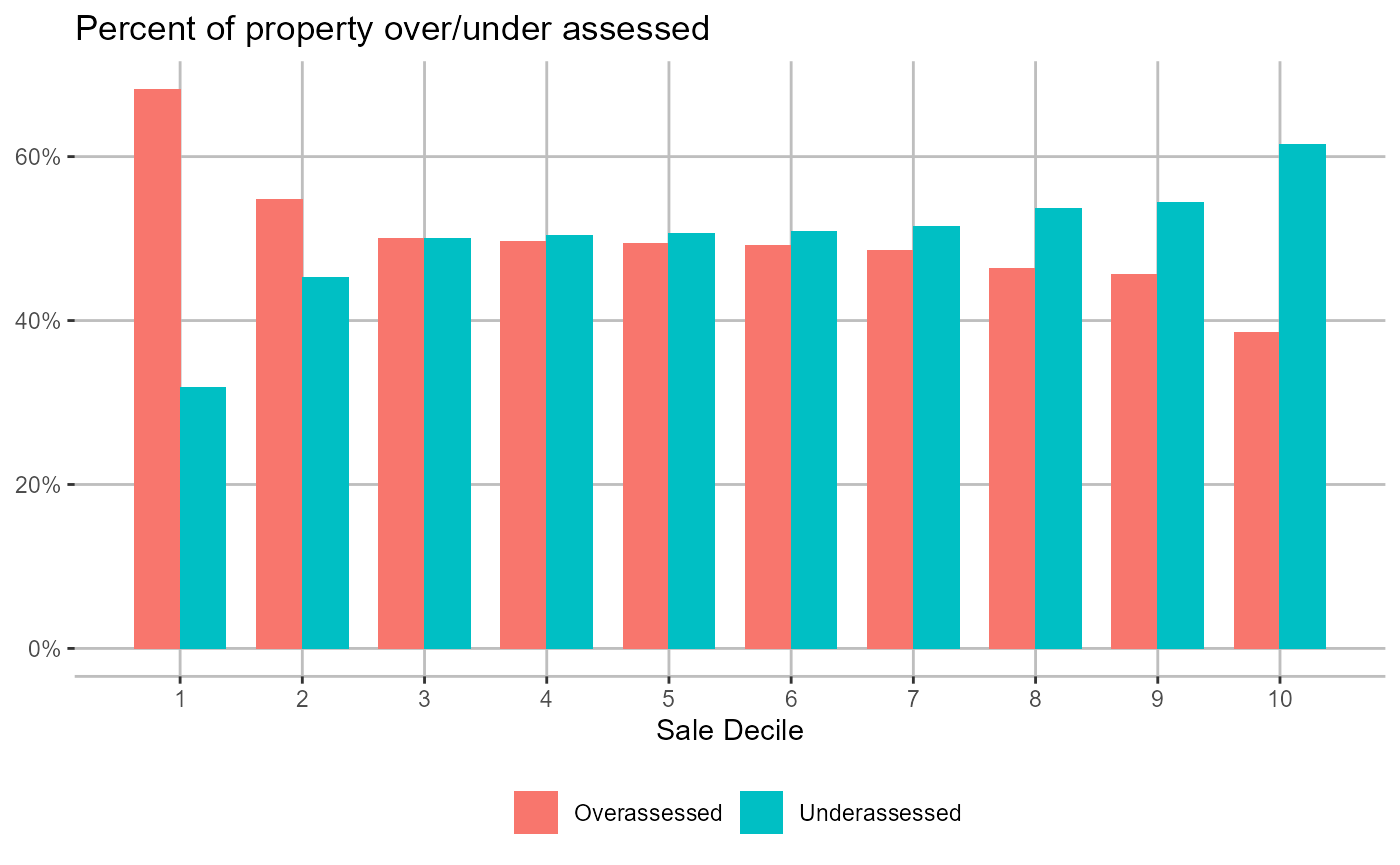This article produces a gallery of figures and tables produced by this package for reference.

library(cmfproperty)

ratios <-
cmfproperty::reformat_data(
data = cmfproperty::example_data,
sale_col = "SALE_PRICE",
assessment_col = "ASSESSED_VALUE",
sale_year_col = "SALE_YEAR",
)
#>  "Filtered out non-arm's length transactions"
#>  "Inflation adjusted to 2019"
stats <- cmfproperty::calc_iaao_stats(ratios)

# calc_iaao_stats

rmarkdown::paged_table(stats)

# regression_tests

summary_info <-
cmfproperty::regression_tests(ratios, produce_table = TRUE)
 Dependent Variable ASSESSED_VALUE log(ASSESSED_VALUE) RATIO (1) (2) (3) SALE_PRICE 0.77*** -0.0000*** (0.001) (0.00) log(SALE_PRICE) 0.91*** (0.001) Constant 34,702.12*** 0.95*** 0.96*** (256.32) (0.01) (0.001) Observations 308,031 308,031 308,031 R2 0.84 0.86 0.03 Adjusted R2 0.84 0.86 0.03 Note: p<0.1; p<0.05; p<0.01
kableExtra::kable(summary_info)
Model Value Test T Statistic Conclusion Model Description
paglin72 34702.1237052 > 0 135.385620 Regressive AV ~ SP
cheng74 0.9136623 < 1 1348.353690 Regressive ln(AV) ~ ln(SP)
IAAO78 -0.0000001 < 0 -97.596795 Regressive RATIO ~ SP
kochin82 0.9359248 < 1 1348.353690 Regressive ln(SP) ~ ln(AV)
bell84 20314.8672457 > 0 77.266036 Regressive AV ~ SP + SP^2
0.0000000 < 0 -157.626702 Regressive AV ~ SP + SP^2
sunderman90 11111.3515478 > 0 5.063213 Regressive AV ~ SP + low + high + low * SP + high * SP

# iaao_graphs

iaao_rslt <-
cmfproperty::iaao_graphs(
stats,
ratios,
min_reporting_yr = 2015,
max_reporting_yr = 2019,
jurisdiction_name = "Cook County, Illinois"
)

## Coefficient of Dispersion (COD)

print(iaao_rslt[])
#>  "For 2019, the COD in Cook County, Illinois was 18.19 which <b>did not meet</b> the IAAO standard for uniformity. "
iaao_rslt[]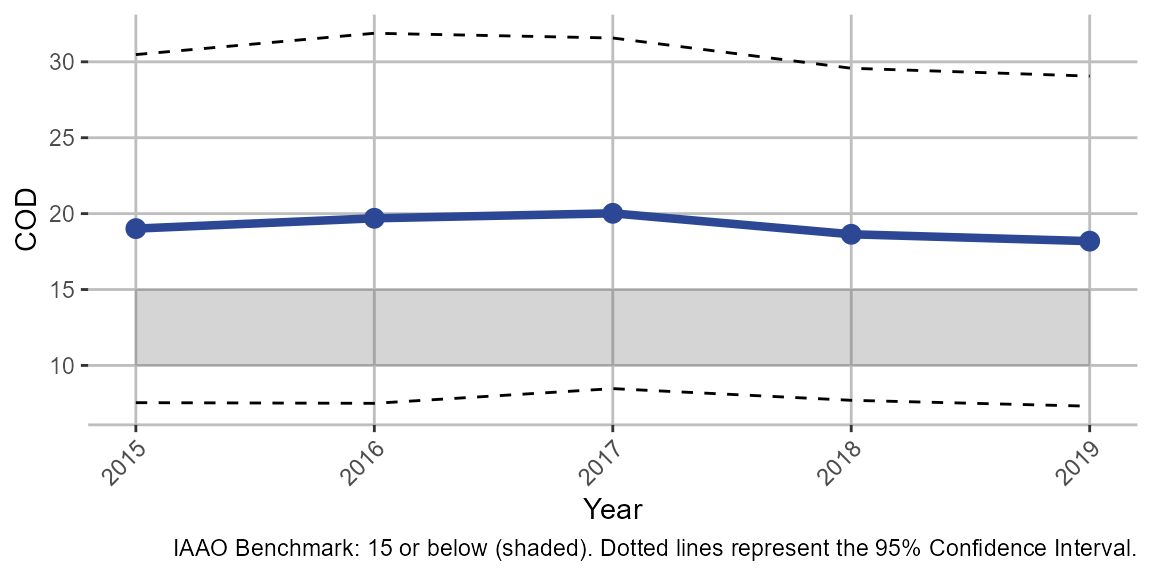# monte_carlo_graphs

m_rslts <- cmfproperty::monte_carlo_graphs(ratios)
gridExtra::grid.arrange(m_rslts[],
m_rslts[],
m_rslts[],
m_rslts[],
m_rslts[],
m_rslts[],
nrow = 3)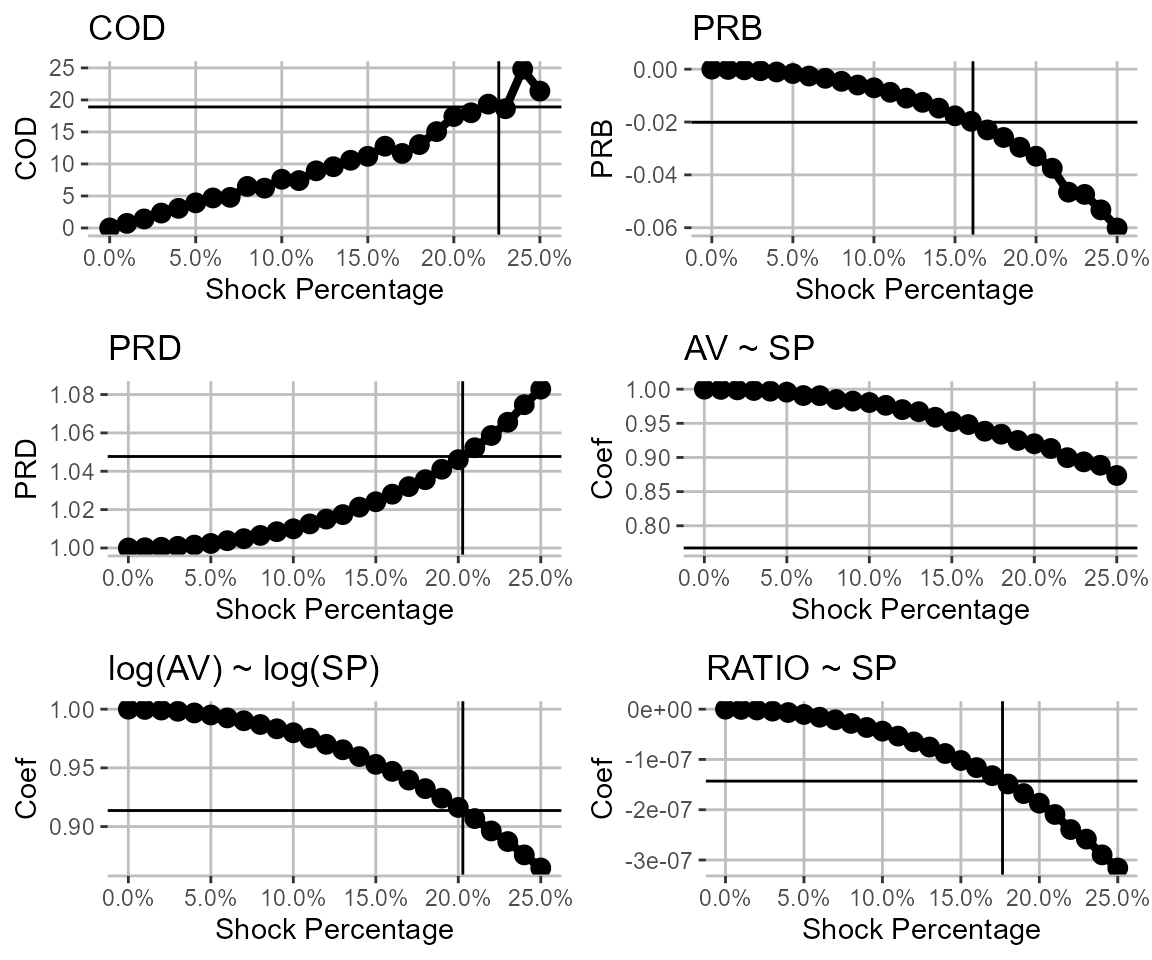# diagnostic_plots

plots <-
diagnostic_plots(stats,
ratios,
min_reporting_yr = 2015,
max_reporting_yr = 2019)
plots[]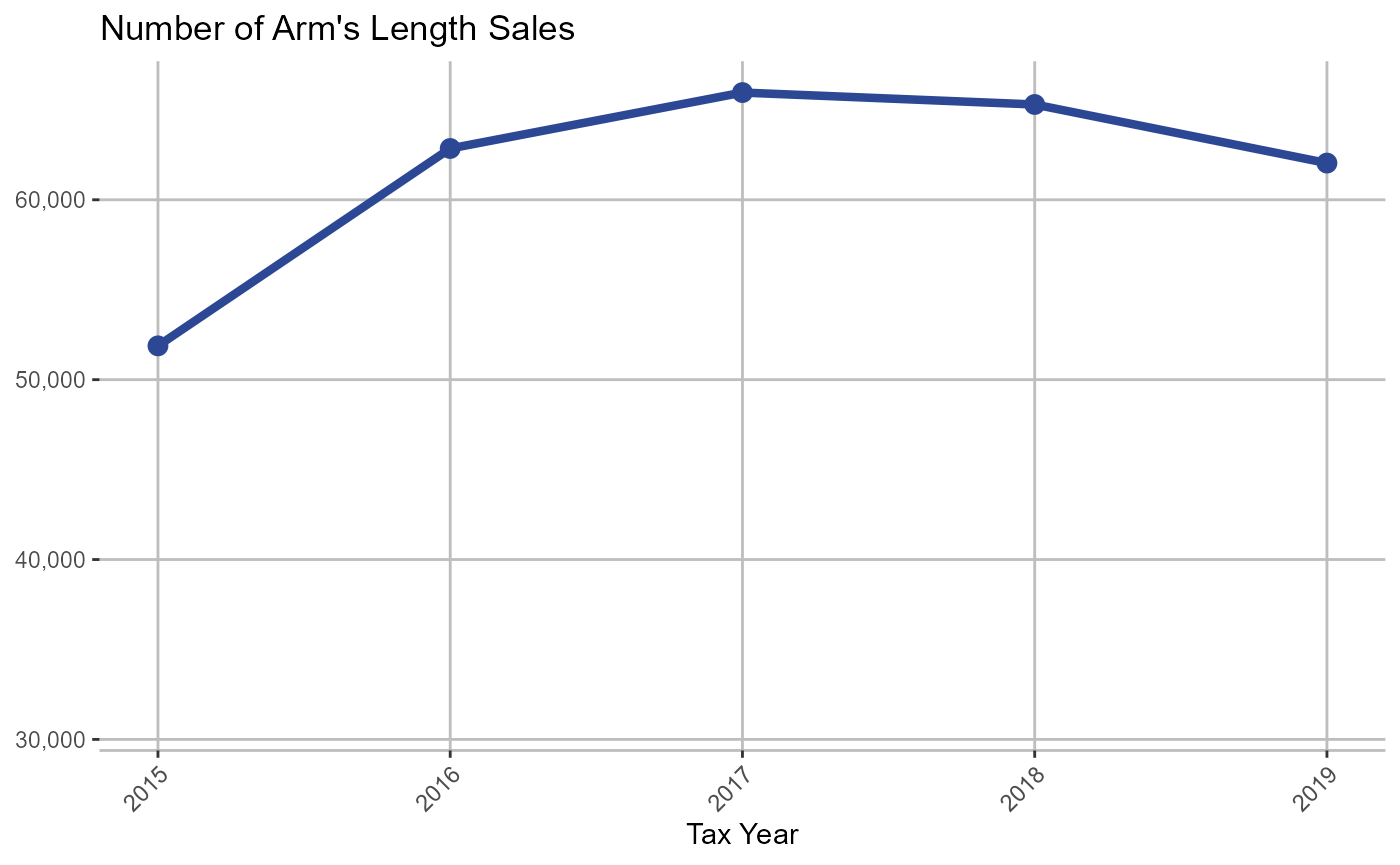plots[]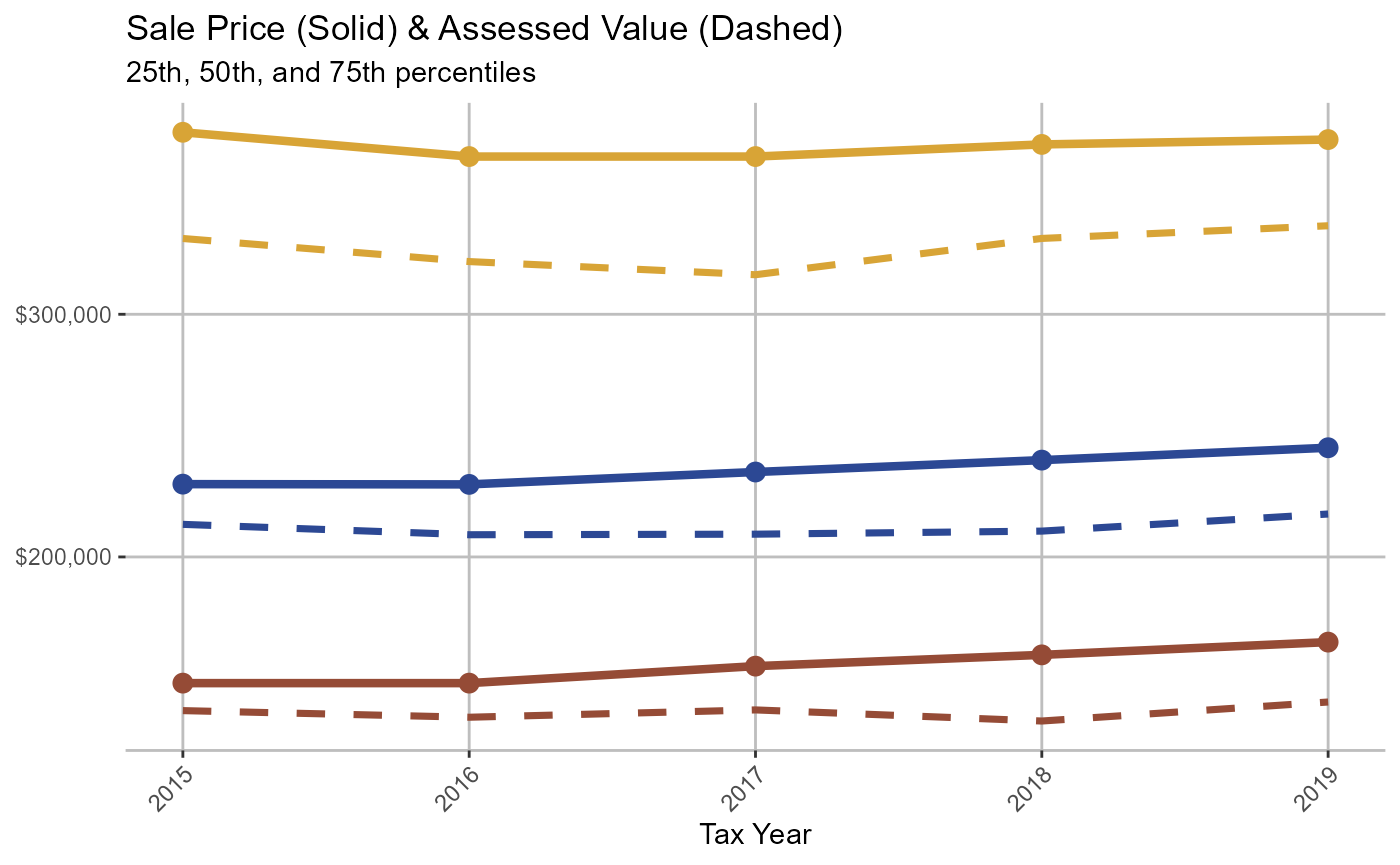plots[]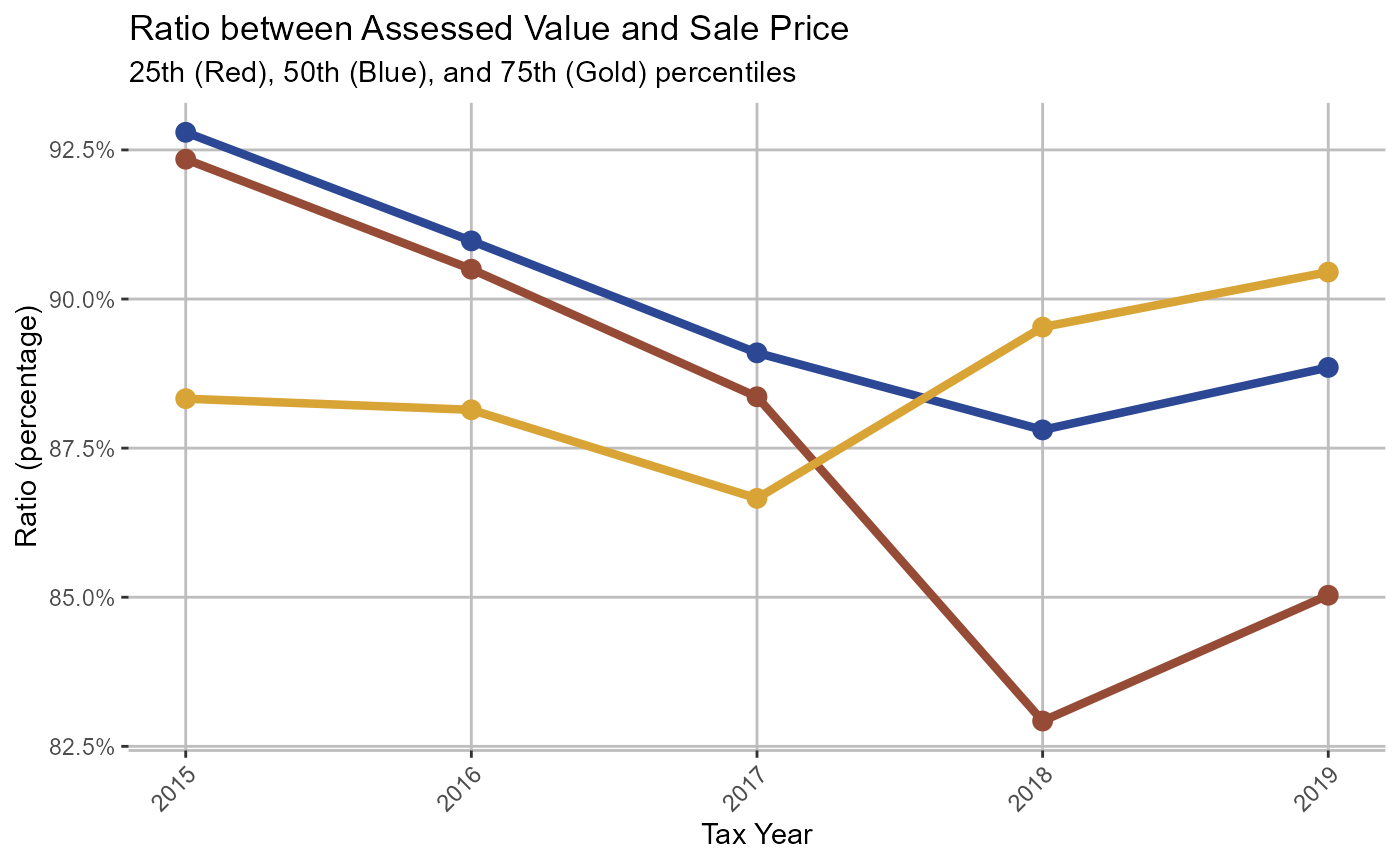plots[]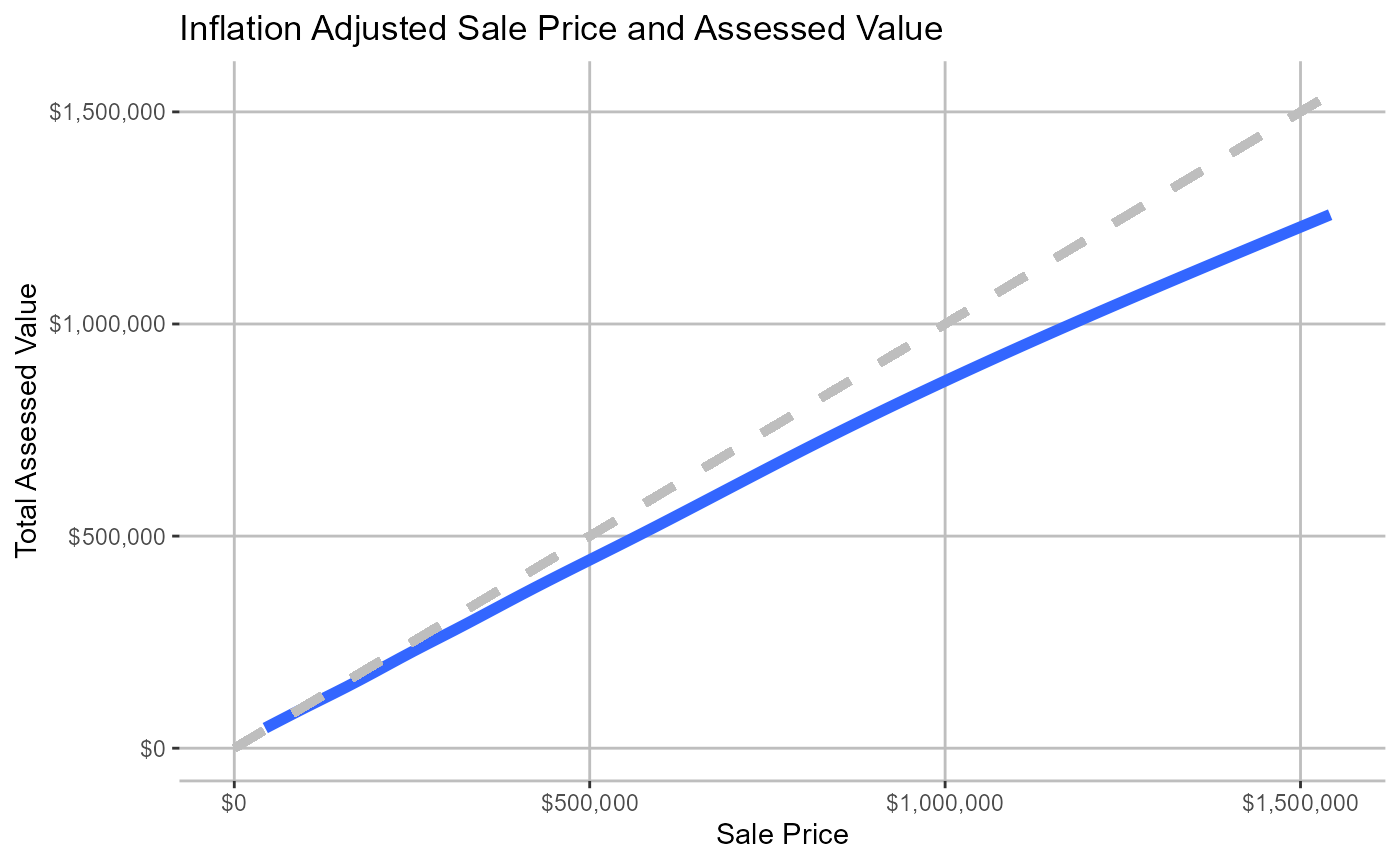plots[]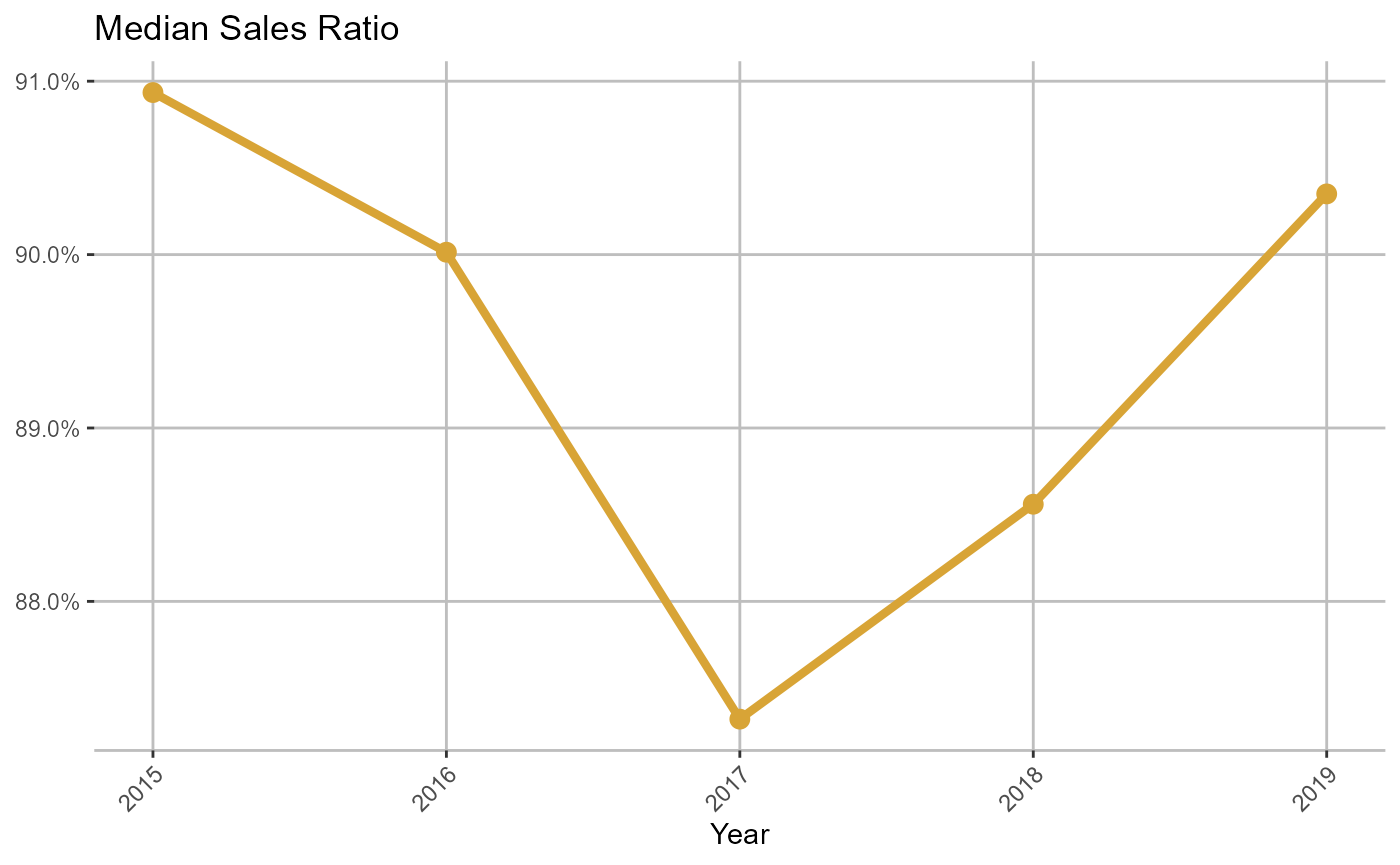gridExtra::grid.arrange(plots[],
plots[],
plots[],
plots[],
ncol = 2,
nrow = 2)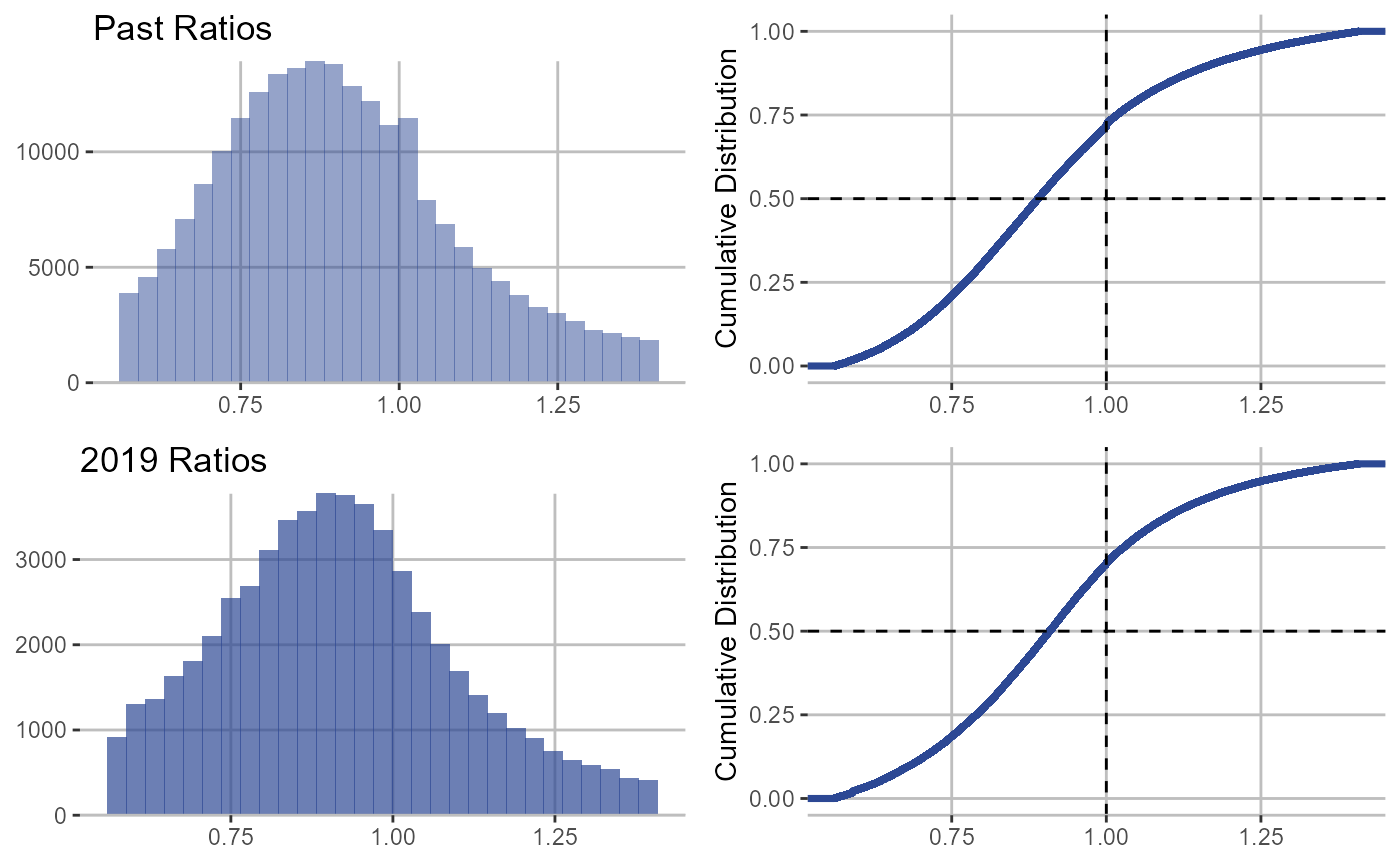# binned_scatter

binned <-
cmfproperty::binned_scatter(
ratios,
min_reporting_yr = 2015,
max_reporting_yr = 2019,
jurisdiction_name = "Cook County, IL"
)

print(binned[])
#>  "In 2019, the most expensive homes (the top decile) were assessed at 87.1% of their value and the least expensive homes (the bottom decile) were assessed at 102.0%. In other words, the least expensive homes were assessed at <b>1.17 times</b> the rate applied to the most expensive homes. Across our sample from 2015 to 2019, the most expensive homes were assessed at 83.4% of their value and the least expensive homes were assessed at 109.4%, which is <b>1.31 times</b> the rate applied to the most expensive homes."
binned[]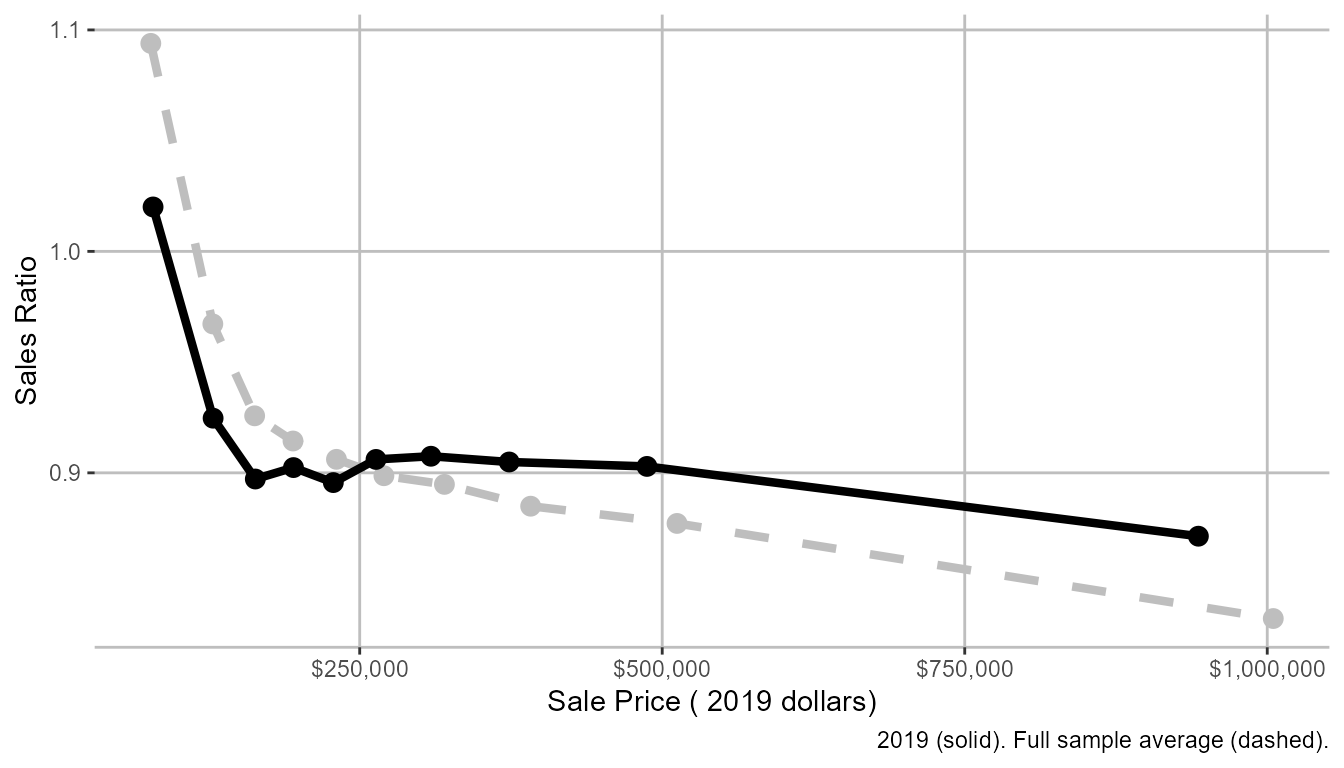# pct_over_under

pct_over <-
cmfproperty::pct_over_under(
ratios,
min_reporting_yr = 2015,
max_reporting_yr = 2019,
jurisdiction_name = "Cook County, IL"
)

print(pct_over[])
#>  "In Cook County, IL, <b>68%</b> of the lowest value homes are overassessed and <b>39%</b> of the highest value homes are overassessed."
pct_over[]